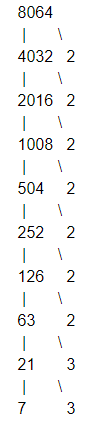Courses
Courses for Kids
Free study material
Offline Centres
MoreLast updated date: 29th Nov 2023
Total views: 384k
Views today: 7.84k

# Find the number of divisors of 8064.Verified
384k+ views
Hint: Apply prime factorisation to find the prime factors of the given number and then apply formula to find the number of divisors of the given number.

The given number is 8064.
First we find all the prime factors of the given number.
Prime factors are numbers which are divisible by itself, that is, it has ‘one’ and itself as the factors.
Now let’s find all the prime factors of 8064 using prime factorisation methodSo, 8064 can be written as,
$8064=2\times 2\times 2\times 2\times 2\times 2\times 2\times 3\times 3\times 7$
By grouping, we get
$8064={{2}^{7}}\times {{3}^{7}}\times {{7}^{1}}.........\left( 1 \right)$
Now to find the number of divisors we will use the formula,
$d\left( n \right)=\left( p+1 \right)\times \left( q+1 \right)\times \left( r+1 \right)$
Where ‘n’ is the number and ‘p, q, r’ are the powers of prime factors of the numbers or they are the exponents of prime factorisation.
Such that a number can be represented as,
$n={{a}^{p}}\times {{b}^{q}}\times {{c}^{r}}.........\left( 2 \right)$
Where ‘a, b, c’ are prime factors of ‘n’
Now applying this formula in our problem, we get
Comparing equation (1) and (2), we get
P=7, q=2, r=1
So the number of divisors will be
$d\left( 8064 \right)=\left( 7+1 \right)\times \left( 2+1 \right)\times \left( 1+1 \right)$
Solving we get
\begin{align} & d\left( 8064 \right)=8\times 3\times 2 \\ & \Rightarrow d\left( 8064 \right)=48 \\ \end{align}
So the number of divisors of 8064 is 48.

Note: Student forgets to add one in the divisor formula i.e.,
$d\left( n \right)=\left( p+1 \right)\times \left( q+1 \right)\times \left( r+1 \right)........\left( A \right)$
Will be mistakenly written as,
$d\left( n \right)=p\times q\times$$r........\left( B \right)$
The answer we get in both cases does not match and the student will end up in the wrong answer if he uses formula (B) instead of (A).